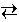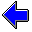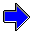# Chemical Equilibria

## Le Châtelier's Principle: Effect of a Change in Volume

### Concepts

Le Châtelier's Principle

When a stress is brought to bear on a system at equilibrium, the system will react in the direction that serves to relieve the stress.

In the previous experiments Le Châtelier's Principle was employed to predict the behavior of a system when the amount of a reactant or product is changed or when the temperature is changed. In this experiment the stress brought upon the system is a change in volume.

What is the consequence of changing the volume of a gas?

The fundamental effect of a change in volume is to simultaneously change the partial pressures (and molar concentrations) of all reactants and products by the same relative amount. If the volume of the gas is reduced, the concentrations of all reactants and products are simultaneously increased, which corresponds with an increase in the total concentration of species in the gas. Using Le Châtelier's Principle, the system should react in a way to oppose this stress; that is, the system should react to reduce the total concentrations.

Note that species that do not participate in a reaction are unaffected by the position of the equilibrium for the reaction. Thus adding an unreactive species, such as nitrogen gas or helium, has no influence upon the reaction, because such species are neither reactants nor products in the reaction. (Strictly speaking, this is true only for systems that behave ideally.)

In addition, the only species that are affected by a change in volume are gas-phase or solution-phase species. (For a solution, the volume change would be accomplished by adding pure solvent or removing pure solvent by evaporation.) Such species are the only type whose concentrations are altered by a volume change.

To illustrate this effect, consider the steam reforming reaction used in previous experiments.

C (s) + H2O (g)CO (g) + H2 (g)

In this experiment the volume of the system can be changed and the corresponding changes in equilibrium amounts of carbon, water, carbon monoxide, and hydrogen can be observed.

### Experiment

Objective:

Explore the effect of a change in volume on the equilibrium composition of a system.

Experiment:

The piston shown below contains carbon, water, carbon monoxide, and hydrogen at 1000.0 K. (The solid carbon is not actually shown in the image.) The scale on the side of the piston shows the volume of the enclosed region in liters. The reset button sets the volume to 10.0 L.

1. Reset the experiment. Drag the piston barrier to increase the volume of the system. What happens to the equilibrium amounts of hydrogen and carbon monoxide in the system? What happens to the equilibrium amounts of carbon and water? Is this behavior consistent with Le Châtelier's Principle? Drag the piston barrier to decrease the volume of the system.

2. Reset the experiment. Use the equilibrium amounts of each species and the system temperature of 1000. K to calculate the equilibrium constant KP at 10.0 L. Drag the piston barrier to change the volume of the system and recalculate the equilibrium constant. Does the equilibrium constant depend upon the volume of the system? (In these calculations, assume the volume occupied by the carbon, which is a solid, is negligible. The volume in liters is shown by the scale at the right of the piston.)

After performing the experiment, carefully think about the results. If the volume of the system is increased, why would the system respond by converting carbon and water into carbon monoxide and hydrogen?

 Equilibrium Amounts of Material in the Glass Bulb C moles           H2O moles           CO moles           H2 molesLe Châtelier's Principle                     Le Châtelier's Principle: Changes in TemperatureChemical Equilibria Home PageVirtual Chemistry Home Page

Volume.html version 2.1
© 2001-2014 David N. Blauch